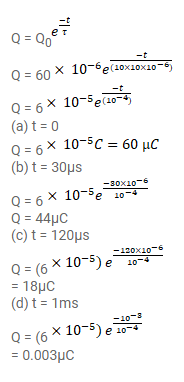# The plates of a capacitor of capacitance 10μF,

Question:

The plates of a capacitor of capacitance $10 \mu \mathrm{F}$, charged to $60 \mu \mathrm{C}$, are joined together by a wire of resistance $10 \Omega$ at $t=0$. Find the charge on the capacitor in the circuit at

(a) $t=0$,

(b) $t=30 \mu \mathrm{s}$,

(c) $t=120 \mu \mathrm{s}$ and

(d) $t=1.0 \mathrm{~ms}$

Solution: